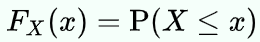# What is a «cumulative distribution function» («CDF»)?

The cumulative distribution function (CDF) of a real-valued random variable X evaluated at x, is the probability that X will take a value ≤ x.en.wikipedia.org/wiki/Cumulative_distribution_function